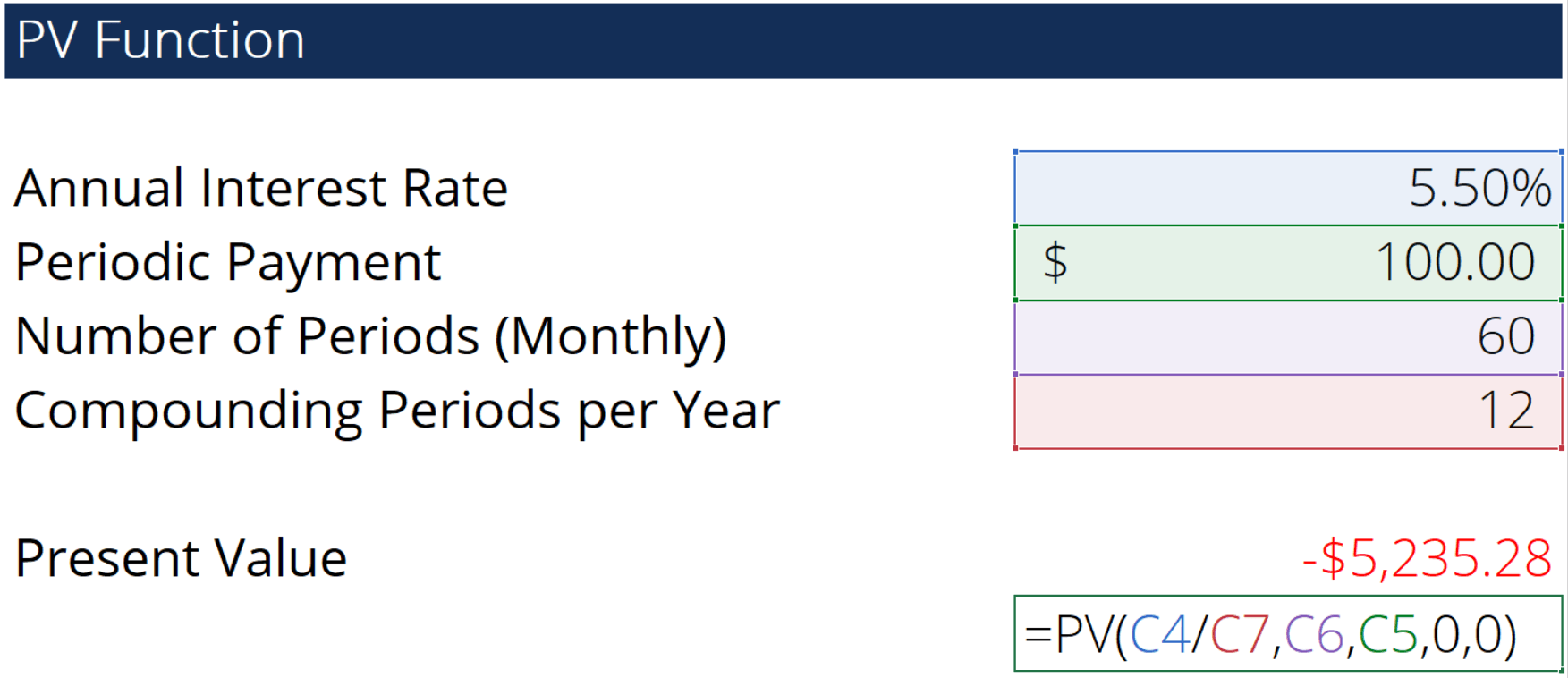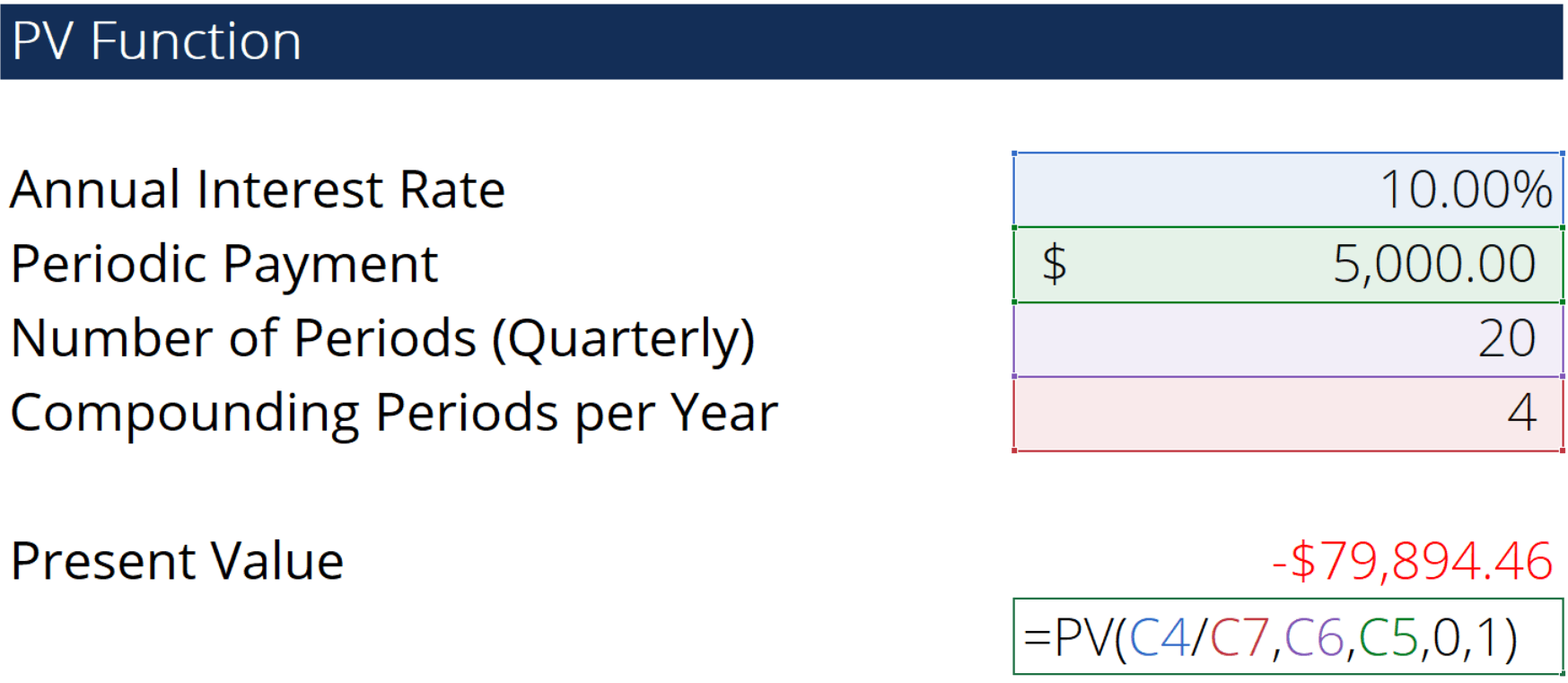# PV Function

Calculates the present value of an investment or a loan taken at a fixed interest rate

## What is the PV Function?

The PV function is a widely used financial function in Microsoft Excel. It calculates the present value of a loan or an investment.

In financial statement analysis, PV is used to calculate the dollar value of future payments in the present time. For multiple payments, we assume periodic, fixed payments and a fixed interest rate. Alternatively, the function can also be used to calculate the present value of a single future value.

### Formula

=PV(rate, nper, pmt, [fv], [type])

The PV function uses the following arguments:

1. rate (required argument) – The interest rate per compounding period. A loan with a 12% annual interest rate and monthly required payments would have a monthly interest rate of 12%/12 or 1%. Therefore, the rate would be 1%.
2. nper (required argument) – The number of payment periods. For example, a 3 year loan with monthly payments would have 36 periods. Therefore, nper would be 36 months.
3. pmt (required argument) – The fixed payment per period.
4. fv (optional argument) – An investment’s future value at the end of all payment periods (nper). If there is no input for fv, Excel will assume the input is 0.
5. type (optional argument) – Type indicates when payments are issued. There are only two inputs, 0 and 1. If type is omitted or 0 is the input, payments are made at period end. If set to 1, payments are made at period beginning.

#### Notes

1. An annuity consists of multiple fixed cash payments made over a specific period (e.g. car loans, mortgages)
2. Cash outflows, such as deposits to a trust fund, are shown negative numbers. Cash inflows, such as dividends on investments, are shown as positive numbers.

### How to use the PV Function in Excel

To understand the uses of the PV function, let us consider a few examples:

#### Example 1

For this example, we have an annuity that pays periodic payments of \$100.00 with a 5.5% annual interest rate. This annuity makes payments on a monthly basis and will do so for 5 years. The setup and formula for the PV function would be as shown below:Using the PV function, we calculate that the fair present value, if you were to purchase this annuity today, would be \$5,235.28.

#### Example 2

Alternatively, we have an annuity that makes periodic payments of \$5000.00 every quarter for 5 years with a 10% interest rate. Payments are made at period beginning. The setup would be as follows:Using this function, we calculate that the fair present value, if were to purchase this annuity today, would be \$79,894.46.

### Things to remember about the PV Function

1. #VALUE! error – Occurs if any of the given arguments is non-numeric.
2. One common error in using the PV function is not converting the annual interest rate into a periodic interest rate, or as payments are made. If omitted, it can result in an error.

### PV Function Template

Thanks for reading CFI’s guide to important Excel functions! By taking the time to learn and master these functions, you’ll significantly speed up your financial modeling and analysis. To learn more, check out these additional CFI resources:

• Excel Functions for Finance
• Types of Graphs in Excel
• All Excel Resources
• Certified Financial Analyst

### Free Excel Tutorial

To master the art of Excel, check out CFI’s FREE Excel Crash Course, which teaches you how to become an Excel power user.  Learn the most important formulas, functions, and shortcuts to become confident in your financial analysis.

Launch CFI’s Free Excel Course now to take your career to the next level and move up the ladder!